CSC 378: Data Structures and Algorithm Analysis

Red-Black Trees

# Balanced BSTs

[ CLR 13 ]

The height of a BST is number of nodes on the longest root-to-leaf path.

Some definitions of height will use the number of edges, instead. It doesn't really matter when doing asymptotic analysis, since the number of nodes and the number of edges differ by only one.

Insert, Delete, and Search take worst-case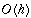in a BST of height h.

A BST of n nodes is balanced if height is in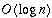. A balanced tree supports efficient operations, since most operations only have to traverse one or two root-to-leaf paths.

There are many implementations of balanced BSTs, including AVL trees, 2/3 trees, and Red-Black trees. We'll discuss Red-Black trees.

# Red-Black Properties

[ CLR 14 ]

Every node in a Red-Black tree stores data (which includes the key), a left child pointer, a right child pointer, a parent pointer, and a ``colour'' attribute. A Red-Black tree satisfies the following properties:

1. Every node is either red or black.
2. If a node has a NULL child, that "child" is considered black.
3. If a node is red, then both of its children are black.
4. Every simple path from a node to a descendant NULL child has the same number of black nodes, (including the black NULL child).
5. The root is black.

Here's an example of a Red-Black tree, where the square black nodes are the ``virtual'' black children of Property II.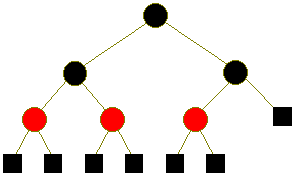# Red-Black Height

These five properties ensure that a Red-Black tree is balanced.

Intuitively: Property IV ensures that a Red-Black tree is balanced if it doesn't contain red nodes, since every root-leaf path has the same number of black nodes. When red nodes are added, Property III ensures that, on a root-to-leaf path with k black nodes, there are at most k red nodes. So adding the red nodes only increases the height by a factor of two.

Let BH(x) be the number of black nodes on every x-to-leaf path. By Property IV, each such path has the same number of black nodes.

For example: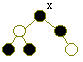BH(x) = 2

Lemma

A subtree rooted at x has at least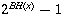nodes.

Intuition

Suppose x's subtree has only black nodes. By Property IV the tree is complete,

For example: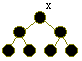BH(x) = 3, number of nodes = 7

and it hasnodes. If red nodes are included, BH(x) doesn't change, so number of nodes is still at least.

Lemma

Let h be the height of the tree. Then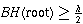.

Intuition

By Property III, each root-to-leaf path has at least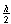black nodes. (Otherwise, two red nodes would appear together, as parent and child.) Thus,.

Theorem

The height, h, of a Red-Black tree is in.

Proof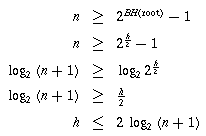Since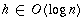, a Red-Black tree is balanced.

The next lecture will discuss insertion into a Red-Black tree.

Back to the lecture index